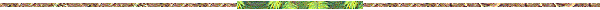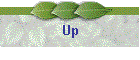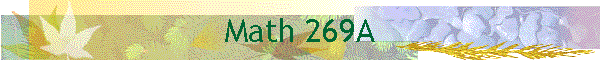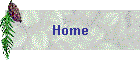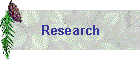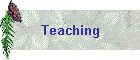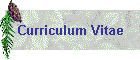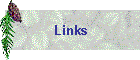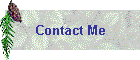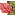Course: Math 269A, Advanced Numerical AnalysisText: A. Iserles, A first course in the Numerical Analysis of Differential Equations", Cambridge Texts in Applied Mathematics, Cambridge University Press, 1998Schedule of Lectures: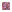Notations and terminology for ODE's and systems of ODE's; reduction of higher order ODE's to 1st order systems of ODE's; the fundamental existence and uniqueness thm. for ODE's (Lipschitz condition)Introduction of Euler's method, order of Euler's method, one step methods (introduction, definition, consistency, local truncation error)Explicit Runge-Kutta (ERK) methods (introduction of the method in the general case, notations in the general case, derivation of ERK of second order); Runge-Kutta method of fourth orderExamples of implicit methods: trapezoidal rule, midpoint rule, the theta method, and the implicit Euler's method; computation of orders for these methodsConvergence of one-step methods (the general case; see also convergence for Euler's method, etc)Asymptotic expansions for the global discretization error for one step methods, and applications to error estimatePractical implementation of one step methodsLinear Multistep methods: examples, derivation using the Lagrange interpolation polynomialLinear multistep methods: definition and computations of the local truncation error, order of the method, consistencyImplicit and explicit linear multistep methods, predictor-corrector methodsExamples of consistent multistep methods which divergeLinear difference equations: stability (root) condition, general solutionConvergence Thm. for linear multistep methodsOrder and consistency for linear multistep methodsAdaptive methods for one-step and multi-step methods, error control, Milne device, extrapolationStiff differential equations, stability and intervals (regions) of absolute stability, A-stable methods, BDF methodsNumerical methods and stability for systems of ODE'sFinite difference methods for linear BVPFunctional (fixed point) iteration and Newton's iteration for solving systems of ODE's using an implicit method